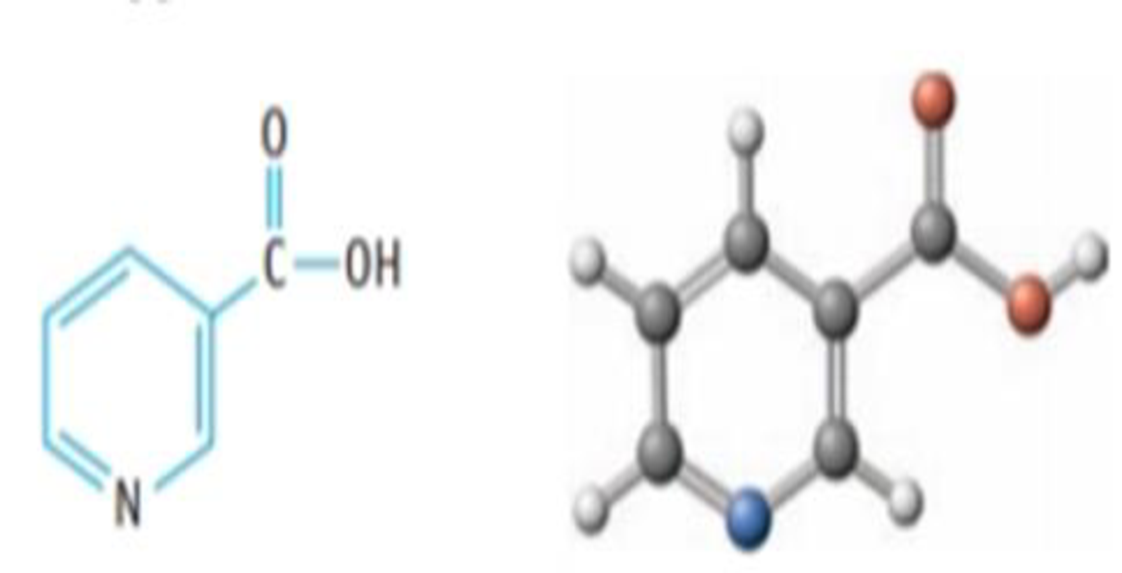# Nicotinic acid, C 6 H 5 NO 2 , is found in minute amounts in all living cells, hut appreciable amounts occur in liver, yeast, milk, adrenal glands, white meat, and corn. Whole wheat (lour contains about 60. 0 μ g per gram of flour. One gram (1.00 g) of the acid dissolves in water to give 60. mL of solution having a pH of 2.70. What is the approximate value of K a for the acid? Nicotinic acid### Chemistry & Chemical Reactivity

9th Edition
John C. Kotz + 3 others
Publisher: Cengage Learning
ISBN: 9781133949640

#### Solutions

Chapter
Section### Chemistry & Chemical Reactivity

9th Edition
John C. Kotz + 3 others
Publisher: Cengage Learning
ISBN: 9781133949640
Chapter 16, Problem 113IL
Textbook Problem
36 views

## Nicotinic acid, C6H5NO2, is found in minute amounts in all living cells, hut appreciable amounts occur in liver, yeast, milk, adrenal glands, white meat, and corn. Whole wheat (lour contains about 60. 0μg per gram of flour. One gram (1.00 g) of the acid dissolves in water to give 60. mL of solution having a pH of 2.70. What is the approximate value of Ka for the acid?Nicotinic acid

Interpretation Introduction

Interpretation:

To calculate the Ka value for the Nicotinic acid.

Concept introduction:

The pH of a solution is measure of hydronium ion concentration in a solution.

pH=log[H3O+]                                                                                                     (1)

The reaction of the Nicotinic acid with water is an example of acid hydrolysis where water acts as a base which abstract proton from the carboxyl part of the Nicotinic acid.

### Explanation of Solution

An equilibrium constant (K) is the ratio of the concentration of products and reactants raised to appropriate stoichiometric coefficient at equilibrium.

The dissociation constant of Nicotinic acid with water is written as follows,

C6H5NO2(aq)+H2O(l)C6H4NO2(aq)+H3O+(aq)

The relative strength of an acid and base in water can be also expressed quantitatively with an equilibrium constant as follows:

Ka=[C6H4NO2][H3O+][C6H5NO2]                                                                                       (2)

An equilibrium constant (K) with subscript a indicates that it is an equilibrium constant of an acid in water.

Given:

The pH of the solution is 2.70.

Mass of Nicotinic acid is 1g.

The Volume of the solution is 0.06L.

The Molecular weight of the Nicotinic acid is 123.11gmol1.

The number of moles (n) is equal to the mass of Nicotinic acid divided by its molecular weight.

n=1g123.11gmol1=0.008mol

The initial concentration of Nicotinic acid (M) is equal to the number of moles (n) divided by the volume of solution.

M=0.008mol0.06L=0.13molL1

From equation (1), calculating the concentration of hydronium ion,

2.70=log[H3O+][H3O+]=0

### Still sussing out bartleby?

Check out a sample textbook solution.

See a sample solution

#### The Solution to Your Study Problems

Bartleby provides explanations to thousands of textbook problems written by our experts, many with advanced degrees!

Get Started

Find more solutions based on key concepts
List three reasons why someone might take a multivitamin supplement that does not exceed 100 percent of the RDA...

Nutrition: Concepts and Controversies - Standalone book (MindTap Course List)

What is physically exchanged during crossing over?

Human Heredity: Principles and Issues (MindTap Course List)

What is a life cycle?

Biology: The Dynamic Science (MindTap Course List)

Two transverse sinusoidal waves combining in a medium are described by the wave functions y1 = 3.00 sin (x + 0....

Physics for Scientists and Engineers, Technology Update (No access codes included)